# NCERT Solutions for Class 7 Maths Chapter 14 Symmetry (EX 14.1) Exercise 14.1

## NCERT Solutions for Class 7 Maths Chapter 14 Symmetry (EX 14.1) Exercise 14.1

CBSE Solutions for Class 7 Maths Chapter 14 Symmetry Exercise 14.1 by Vedantu helps students to score high marks in examinations. The solutions are prepared by professors and teachers with vast experience. These solutions strictly follow CBSE guidelines. The solutions are written in simple language for a better understanding of the different concepts. One who studies the solutions of Vedantu is sure to score high marks in the examination. Download Class 7 Maths Chapter 14.1 PDF for free. Every NCERT Solution is provided to make the study simple and interesting on Vedantu. Subjects like Science, Maths, English will become easy to study if you have access to NCERT Solution for Class 7 Science, Maths solutions and solutions of other subjects.

Do you need help with your Homework? Are you preparing for Exams?
Study without Internet (Offline)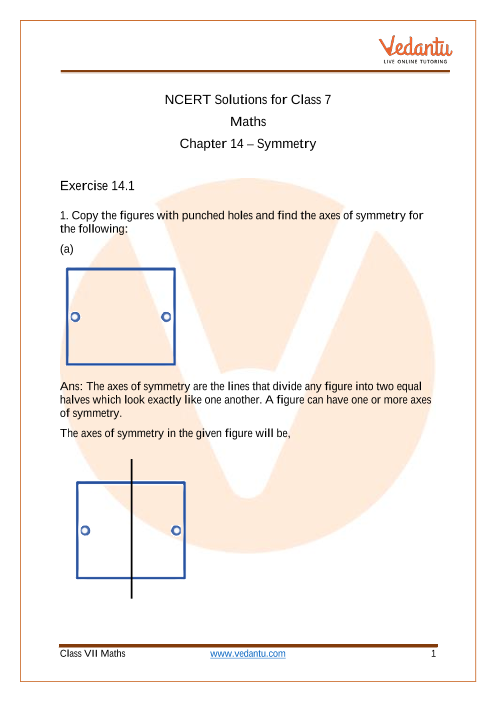Book your Free Demo session
Get a flavour of LIVE classes here at VedantuVedantu Improvement Promise
We promise improvement in marks or get your fees back. T&C Apply*

## Access NCERT Solutions for Class 7 Chapter 14 – Symmetry Exercise 14.1

Exercise 14.1

1. Copy the figures with punched holes and find the axes of symmetry for the following:

(a).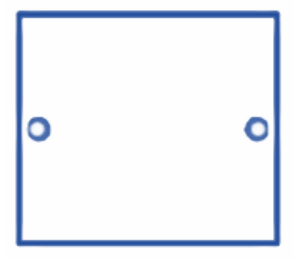Ans: The axes of symmetry are the lines that divide any figure into two equal halves which look exactly like one another. A figure can have more than one axes of symmetry.

The axes of symmetry in the given figure will be,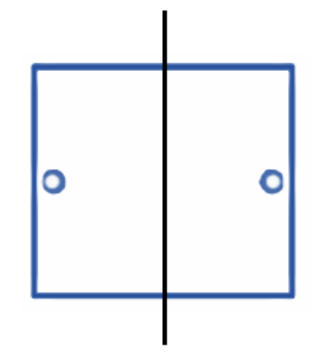(b).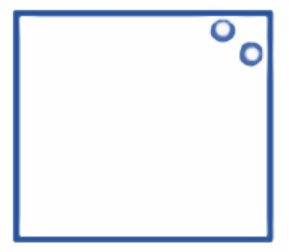Ans: The axes of symmetry are the lines that divide any figure into two equal halves which look exactly like one another. A figure can have more than one axes of symmetry.

The axes of symmetry in the given figure will be,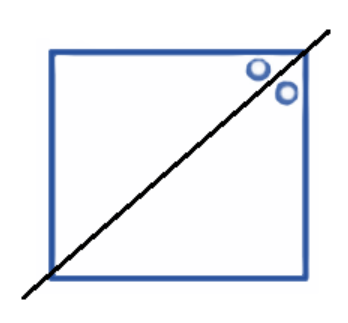(c).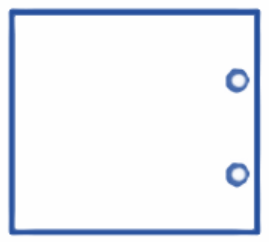Ans: The axes of symmetry are the lines that divide any figure into two equal halves which look exactly like one another. A figure can have more than one axes of symmetry.

The axes of symmetry in the given figure will be,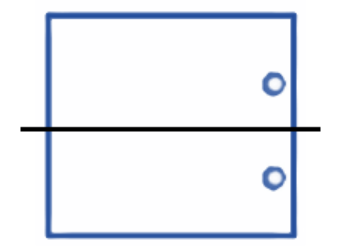(d).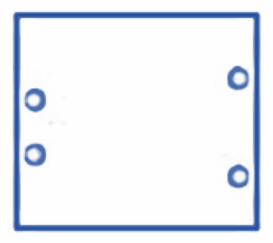Ans: The axes of symmetry are the lines that divide any figure into two equal halves which look exactly like one another. A figure can have more than one axes of symmetry.

The axes of symmetry in the given figure will be,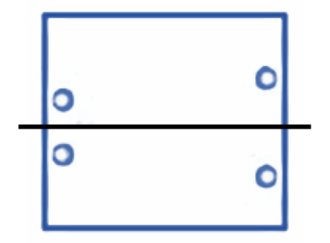(e).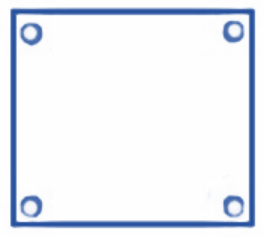Ans: The axes of symmetry are the lines that divide any figure into two equal halves which look exactly like one another. A figure can have more than one axes of symmetry.

The axes of symmetry in the given figure will be,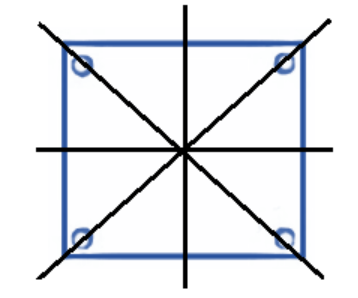(f).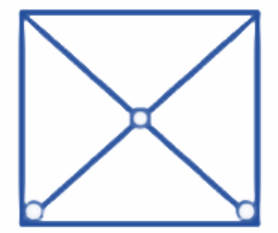Ans: The axes of symmetry are the lines that divide any figure into two equal halves which look exactly like one another. A figure can have more than one axes of symmetry.

The axes of symmetry in the given figure will be,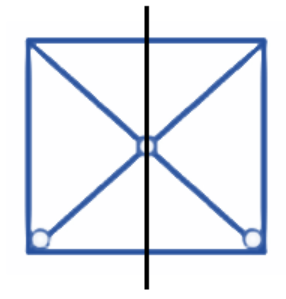(g).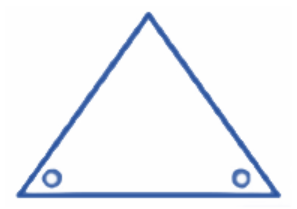Ans: The axes of symmetry are the lines that divide any figure into two equal halves which look exactly like one another. A figure can have more than one axes of symmetry.

The axes of symmetry in the given figure will be,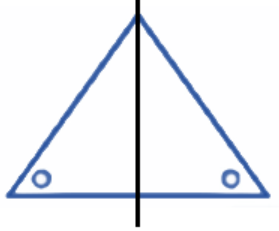(h).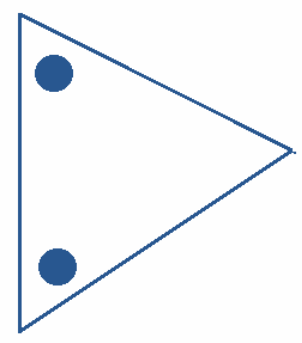Ans: The axes of symmetry are the lines that divide any figure into two equal halves which look exactly like one another. A figure can have more than one axes of symmetry.

The axes of symmetry in the given figure will be,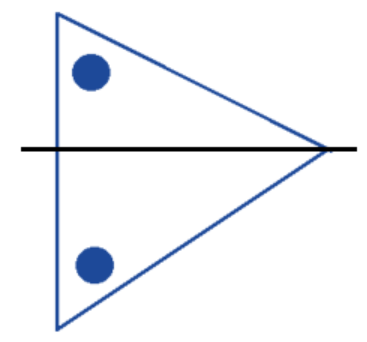(i).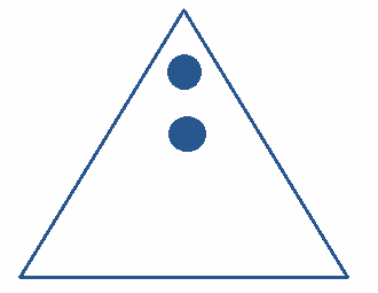Ans: The axes of symmetry are the lines that divide any figure into two equal halves which look exactly like one another. A figure can have more than one axes of symmetry.

The axes of symmetry in the given figure will be,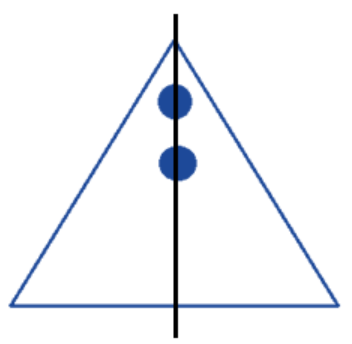(j).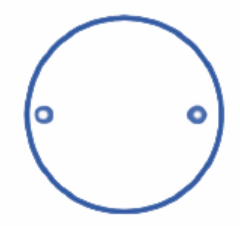Ans: The axes of symmetry are the lines that divide any figure into two equal halves which look exactly like one another. A figure can have more than one axes of symmetry.

The axes of symmetry in the given figure will be,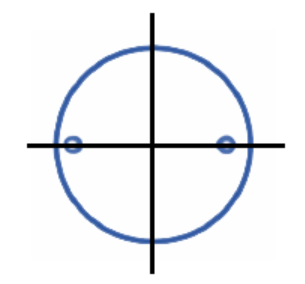(k).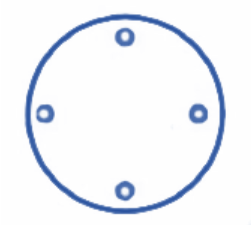Ans: The axes of symmetry are the lines that divide any figure into two equal halves which look exactly like one another. A figure can have more than one axes of symmetry.

The axes of symmetry in the given figure will be,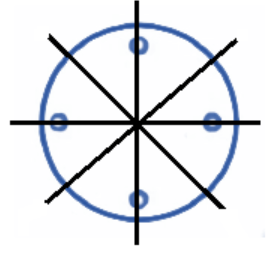(l)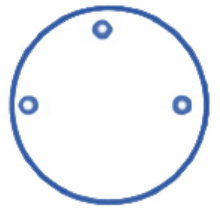Ans: The axes of symmetry are the lines that divide any figure into two equal halves which look exactly like one another. A figure can have more than one axes of symmetry.

The axes of symmetry in the given figure will be,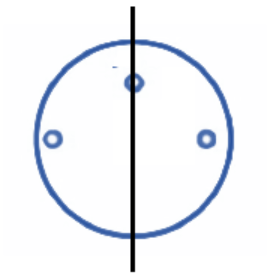2. Given the line(s) of symmetry, find the other hole(s):

(a).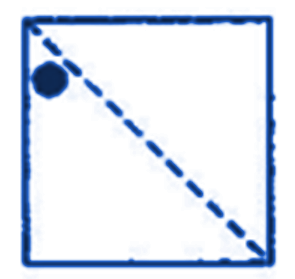Ans: The axes or lines of symmetry are the lines that divide any figure into two equal halves which look exactly like one another. A figure can have more than one axes of symmetry.

The other hole in the given figure along the line of symmetry will be,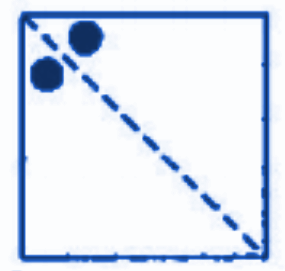(b).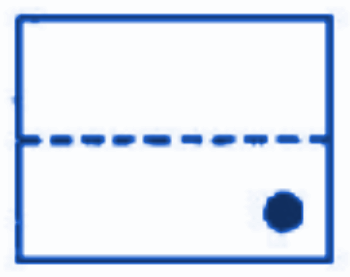Ans: The axes or lines of symmetry are the lines that divide any figure into two equal halves which look exactly like one another. A figure can have more than one axes of symmetry.

The other hole in the given figure along the line of symmetry will be,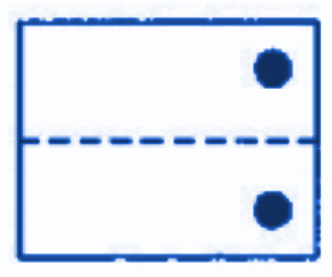(c).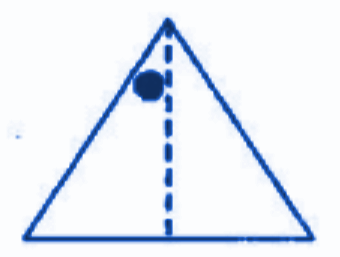Ans: The axes or lines of symmetry are the lines that divide any figure into two equal halves which look exactly like one another. A figure can have more than one axes of symmetry.

The other hole in the given figure along the line of symmetry will be,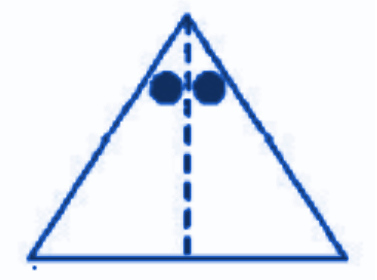(d).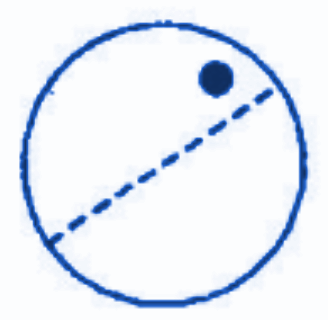Ans: The axes or lines of symmetry are the lines that divide any figure into two equal halves which look exactly like one another. A figure can have more than one axes of symmetry.

The other hole in the given figure along the line of symmetry will be,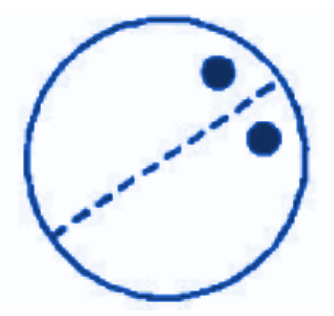(e).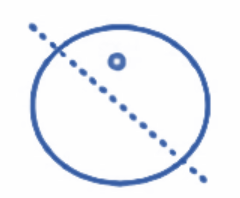Ans: The axes or lines of symmetry are the lines that divide any figure into two equal halves which look exactly like one another. A figure can have more than one axes of symmetry.

The other hole in the given figure along the line of symmetry will be,3. In the following figures, the mirror line (i.e., the line of symmetry) is given as a dotted line. Complete each figure by performing reflection in the dotted (mirror) line. (You might perhaps place a mirror along the dotted line and look into the mirror for the image). Are you able to recall the name of the figure you completed?

(a).Ans: The axes or lines of symmetry or the mirror lines are the lines that divide any figure into two equal halves which look exactly like one another. A figure can have more than one axes of symmetry.

The completed figure after performing the reflection along the dotted mirror line is as follows,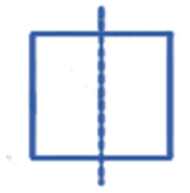The name of the completed figure is square.

(b).Ans: The axes or lines of symmetry or the mirror lines are the lines that divide any figure into two equal halves which look exactly like one another. A figure can have more than one axes of symmetry.

The completed figure after performing the reflection along the dotted mirror line is as follows,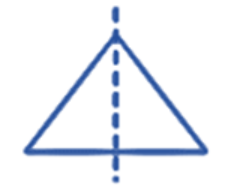The name of the completed figure is triangle.

(c).Ans: The axes or lines of symmetry or the mirror lines are the lines that divide any figure into two equal halves which look exactly like one another. A figure can have more than one axes of symmetry.

The completed figure after performing the reflection along the dotted mirror line is as follows,The name of the completed figure is rhombus.

(d).Ans: The axes or lines of symmetry or the mirror lines are the lines that divide any figure into two equal halves which look exactly like one another. A figure can have more than one axes of symmetry.

The completed figure after performing the reflection along the dotted mirror line is as follows,The name of the completed figure is circle.

(e).Ans: The axes or lines of symmetry or the mirror lines are the lines that divide any figure into two equal halves which look exactly like one another. A figure can have more than one axes of symmetry.

The completed figure after performing the reflection along the dotted mirror line is as follows,The name of the completed figure is pentagon.

(f).Ans: The axes or lines of symmetry or the mirror lines are the lines that divide any figure into two equal halves which look exactly like one another. A figure can have more than one axes of symmetry.

The completed figure after performing the reflection along the dotted mirror line is as follows,The name of the completed figure is octagon.

4. The following figures have more than one line of symmetry. Such figures are said to have multiple lines of symmetry.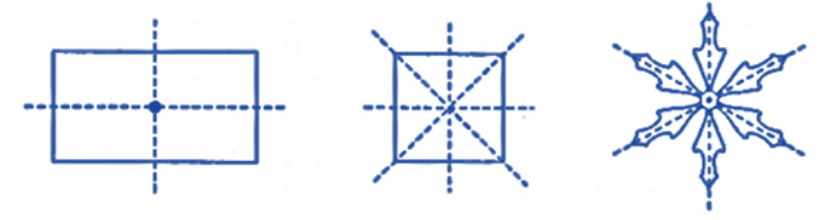Identify multiple lines of symmetry, if any, in each of the following figures:

(a).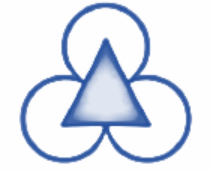Ans: The axes or lines of symmetry or the mirror lines are the lines that divide any figure into two equal halves which look exactly like one another. A figure can have more than one axes of symmetry.

The multiple lines of symmetry in the given figure are as follows,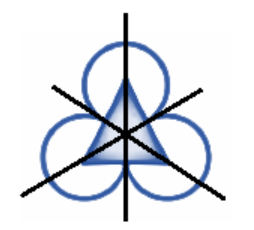(b).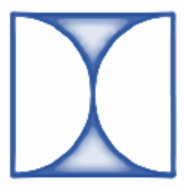Ans: The axes or lines of symmetry or the mirror lines are the lines that divide any figure into two equal halves which look exactly like one another. A figure can have more than one axes of symmetry.

The multiple lines of symmetry in the given figure are as follows,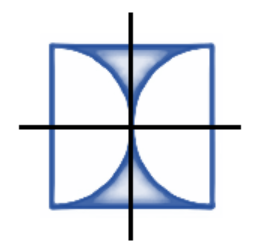(c).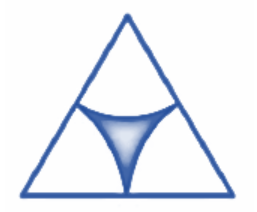Ans: The axes or lines of symmetry or the mirror lines are the lines that divide any figure into two equal halves which look exactly like one another. A figure can have more than one axes of symmetry.

The multiple lines of symmetry in the given figure are as follows,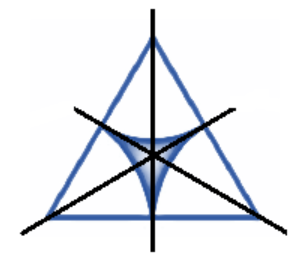(d).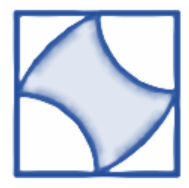Ans: The axes or lines of symmetry or the mirror lines are the lines that divide any figure into two equal halves which look exactly like one another. A figure can have more than one axes of symmetry.

The multiple lines of symmetry in the given figure are as follows,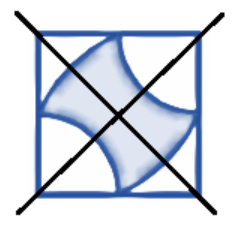(e).Ans: The axes or lines of symmetry or the mirror lines are the lines that divide any figure into two equal halves which look exactly like one another. A figure can have more than one axes of symmetry.

The multiple lines of symmetry in the given figure are as follows,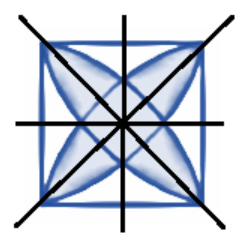(f).Ans: The axes or lines of symmetry or the mirror lines are the lines that divide any figure into two equal halves which look exactly like one another. A figure can have more than one axes of symmetry.

The multiple lines of symmetry in the given figure are as follows,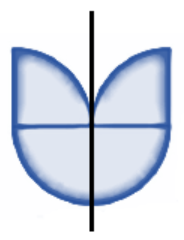(g).Ans: The axes or lines of symmetry or the mirror lines are the lines that divide any figure into two equal halves which look exactly like one another. A figure can have more than one axes of symmetry.

The multiple lines of symmetry in the given figure are as follows,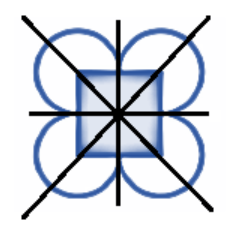(h).Ans: The axes or lines of symmetry or the mirror lines are the lines that divide any figure into two equal halves which look exactly like one another. A figure can have more than one axes of symmetry.

The multiple lines of symmetry in the given figure are as follows,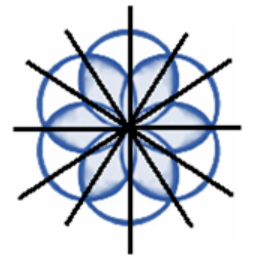5. Copy the figure given here: Take any one diagonal as a line of symmetry and shade a few more squares to make the figure symmetric about a diagonal. Is there more than one way to do that? Will the figure be symmetric about both the diagonals?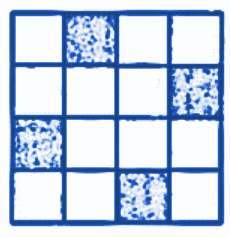Ans: The axes or lines of symmetry or the mirror lines are the lines that divide any figure into two equal halves which look exactly like one another. A figure can have more than one axes of symmetry.

On taking one diagonal as the line of symmetry and shading a few more squares to make the figure symmetric about the diagonal, we obtain the following figure,

On taking one diagonal as the line of symmetry and shading a few more squares to make the figure symmetric about the diagonal, we obtain the following figure,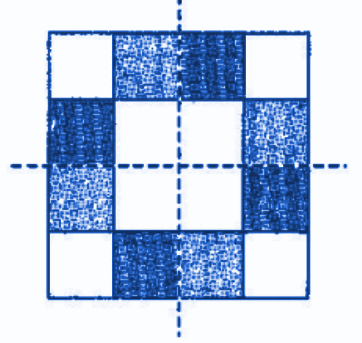Therefore, we can conclude that there is more than one way to make the figure symmetric about the diagonal. Also, we can see that the figure obtained is symmetric about both the diagonals.

6. Copy the diagram and complete each shape to be symmetric about the mirror line(s):

(a).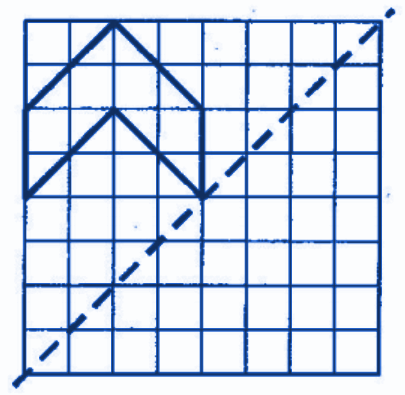Ans: The axes or lines of symmetry or the mirror lines are the lines that divide any figure into two equal halves which look exactly like one another. A figure can have more than one axes of symmetry.

The completed figure after performing the reflection along the dotted mirror line is as follows,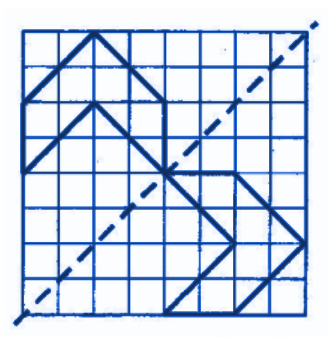(b).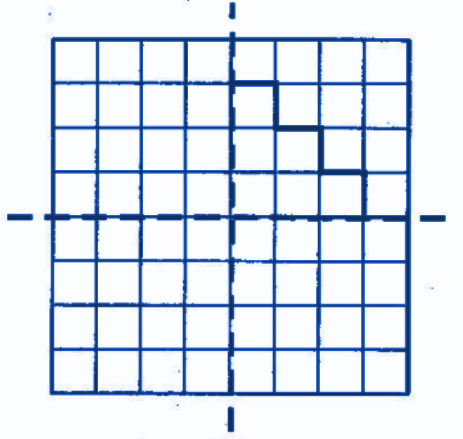Ans: The axes or lines of symmetry or the mirror lines are the lines that divide any figure into two equal halves which look exactly like one another. A figure can have more than one axes of symmetry.

The completed figure after performing the reflection along the dotted mirror line is as follows,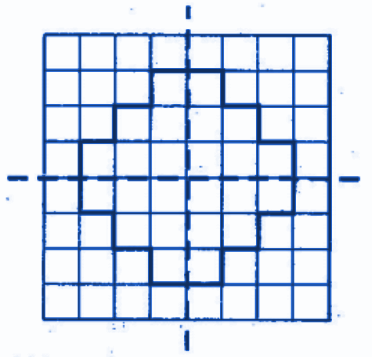(c).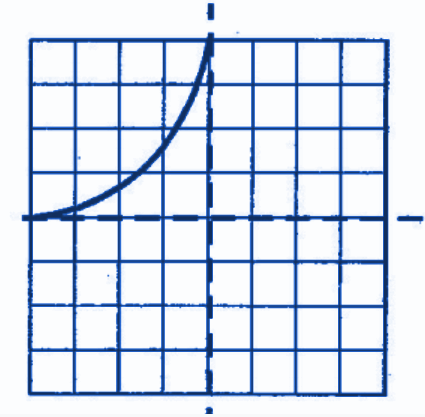Ans: The axes or lines of symmetry or the mirror lines are the lines that divide any figure into two equal halves which look exactly like one another. A figure can have more than one axes of symmetry.

The completed figure after performing the reflection along the dotted mirror line is as follows,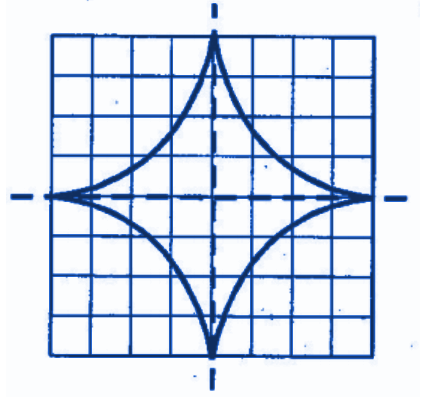(d).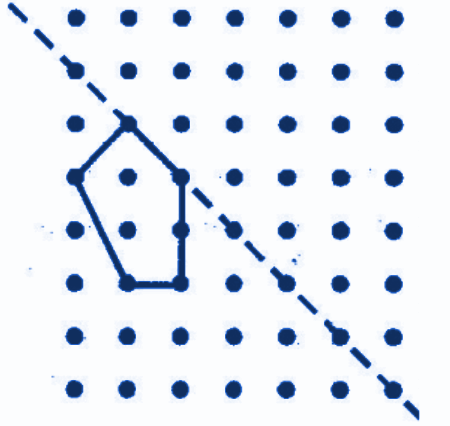Ans: The axes or lines of symmetry or the mirror lines are the lines that divide any figure into two equal halves which look exactly like one another. A figure can have more than one axes of symmetry.

The completed figure after performing the reflection along the dotted mirror line is as follows,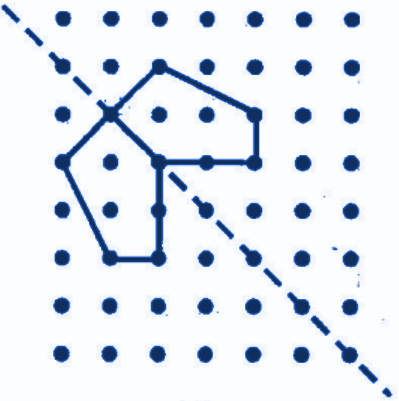7.  State the number of lines of symmetry for the following figures:

(a). An equilateral triangle

Ans: The axes or lines of symmetry or the mirror lines are the lines that divide any figure into two equal halves which look exactly like one another. A figure can have more than one axes of symmetry.

Draw an equilateral triangle. Draw all the possible lines of symmetry for the equilateral triangle.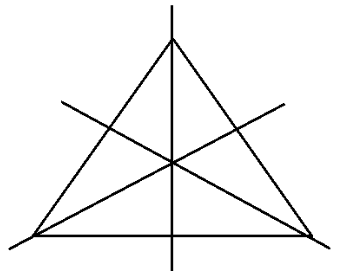There are three lines of symmetry in an equilateral triangle.

(b). An isosceles triangle

The axes or lines of symmetry or the mirror lines are the lines that divide any figure into two equal halves which look exactly like one another. A figure can have more than one axes of symmetry.

Draw an isosceles triangle. Draw all the possible lines of symmetry for the isosceles triangle.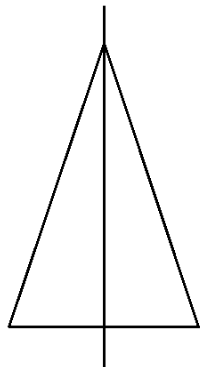There is only one line of symmetry.

(c). A scalene triangle

Ans: The axes or lines of symmetry or the mirror lines are the lines that divide any figure into two equal halves which look exactly like one another. A figure can have more than one axes of symmetry.

Draw a scalene triangle. Draw all the possible lines of symmetry for the scalene triangle.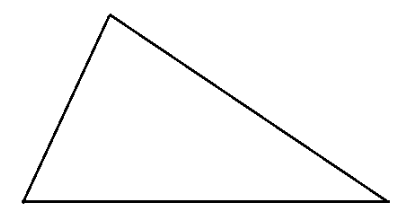There is only no line of symmetry.

(d). A square

Ans: The axes or lines of symmetry or the mirror lines are the lines that divide any figure into two equal halves which look exactly like one another. A figure can have more than one axes of symmetry.

Draw a square. Draw all the possible lines of symmetry for the square.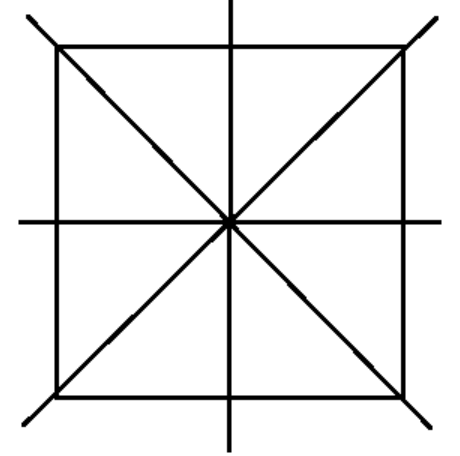There are four lines of symmetry.

(e). A rectangle

Ans: The axes or lines of symmetry or the mirror lines are the lines that divide any figure into two equal halves which look exactly like one another. A figure can have more than one axes of symmetry.

Draw a rectangle. Draw all the possible lines of symmetry for the rectangle.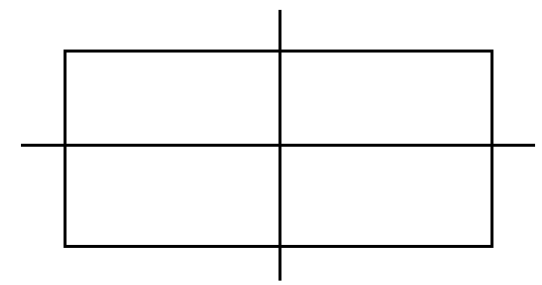There are two lines of symmetry.

(f). A rhombus

Ans: The axes or lines of symmetry or the mirror lines are the lines that divide any figure into two equal halves which look exactly like one another. A figure can have more than one axes of symmetry.

Draw a rhombus. Draw all the possible lines of symmetry for the rhombus.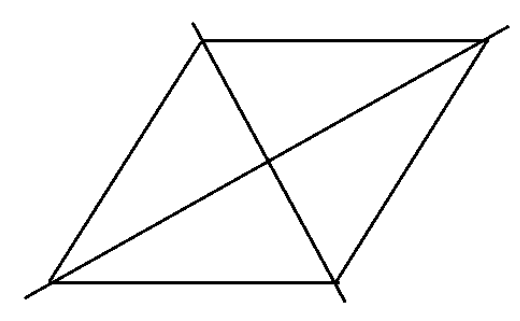There are two lines of symmetry.

(g). A parallelogram

Ans: The axes or lines of symmetry or the mirror lines are the lines that divide any figure into two equal halves which look exactly like one another. A figure can have more than one axes of symmetry.

Draw a parallelogram. Draw all the possible lines of symmetry for the parallelogram.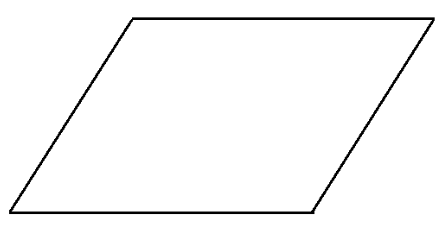There are no lines of symmetry.

Ans: The axes or lines of symmetry or the mirror lines are the lines that divide any figure into two equal halves which look exactly like one another. A figure can have more than one axes of symmetry.

Draw a quadrilateral. Draw all the possible lines of symmetry for the quadrilateral.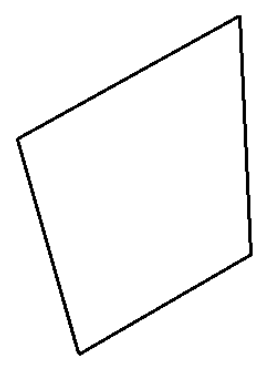There are no lines of symmetry.

(i). A regular hexagon

Ans: The axes or lines of symmetry or the mirror lines are the lines that divide any figure into two equal halves which look exactly like one another. A figure can have more than one axes of symmetry.

Draw a regular hexagon. Draw all the possible lines of symmetry for the regular hexagon.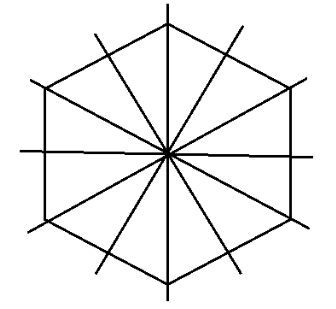There are six lines of symmetry.

(j). A circle

Ans: The axes or lines of symmetry or the mirror lines are the lines that divide any figure into two equal halves which look exactly like one another. A figure can have more than one axes of symmetry.

Draw a circle. Draw all the possible lines of symmetry for the circle.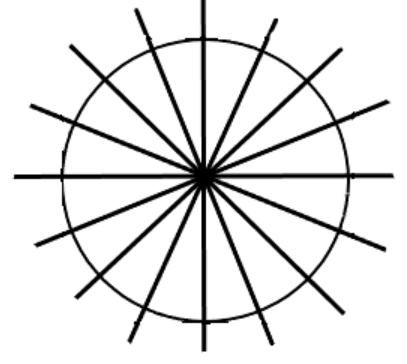There are infinite lines of symmetry.

8. What letters of the English alphabet have reflectional symmetry (i.e., symmetry related to mirror reflection) about:

(a). A Vertical Mirror

Ans: Reflectional symmetry is the symmetry that is caused by the reflections of the mirror. The mirror is placed in different positions like vertically or horizontally to check the reflections and determine whether they are symmetrical or not.

We will place the mirror vertically and check for all the alphabets. On checking, the following alphabets were obtained that were the reflections on the mirror.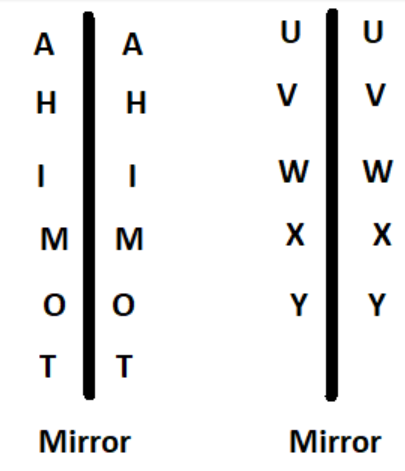(ii) A Horizontal Mirror

Ans: Reflectional symmetry is the symmetry that is caused by the reflections of the mirror. The mirror is placed in different positions like vertically or horizontally to check the reflections and determine whether they are symmetrical or not.

We will place the mirror horizontally and check for all the alphabets. On checking, the following alphabets were obtained that were the reflections on the mirror.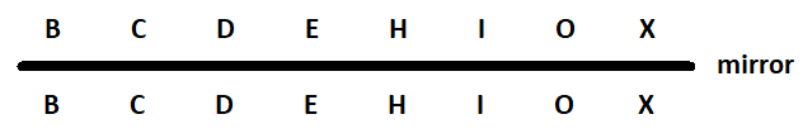(iii) Both Horizontal and Vertical Mirrors

Ans: Reflectional symmetry is the symmetry that is caused by the reflections of the mirror. The mirror is placed in different positions like vertically or horizontally to check the reflections and determine whether they are symmetrical or not.

The alphabets that possess both vertical and horizontal reflections can be determined by placing the mirror once vertically and then horizontally and bringing out the common alphabets from both the cases.

We will place the mirror vertically and check for all the alphabets. On checking the following alphabets were obtained that were the reflections on the mirror.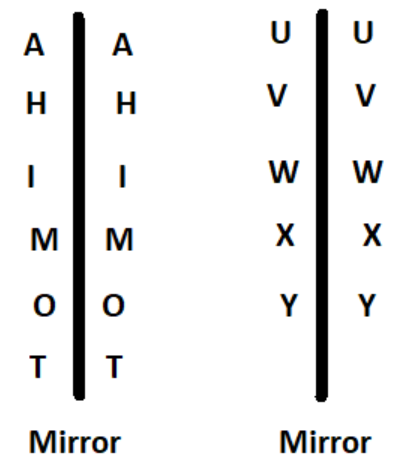We will place the mirror horizontally and check for all the alphabets. On checking the following alphabets were obtained that were the reflections on the mirror.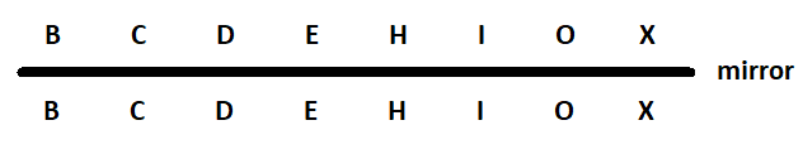From the above two cases we can determine the alphabets that are common for both the vertical and the horizontal reflections.

These alphabets are H, I, O, and X.

9. Give three examples of shapes with no line of symmetry.

The axes or lines of symmetry or the mirror lines are the lines that divide any figure into two equal halves which look exactly like one another. A figure can have more than one axes of symmetry.

Examples of three shapes that have no lines of symmetry are as follows,

1. A Scalene Triangle

A scalene triangle has no lines of symmetry.A quadrilateral has no lines of symmetry.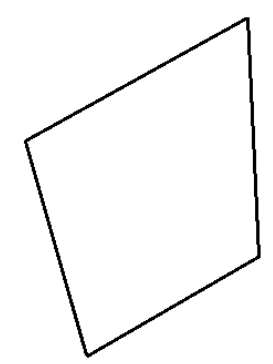1. A Parallelogram

A parallelogram has no lines of symmetry.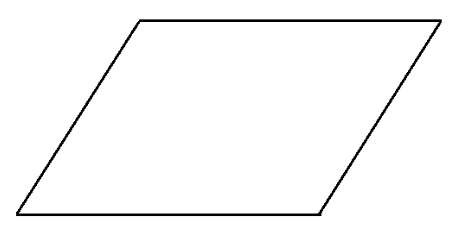10. What other name can you give to the line of symmetry of:

(i) An Isosceles Triangle?

Ans: The axes or lines of symmetry or the mirror lines are the lines that divide any figure into two equal halves which look exactly like one another. A figure can have more than one axes of symmetry.

An isosceles triangle has two equal sides.There is only one line of symmetry. This line of symmetry is also known as the median or the altitude of the triangle.

(ii) A Circle?

Ans: The axes or lines of symmetry or the mirror lines are the lines that divide any figure into two equal halves which look exactly like one another. A figure can have more than one axes of symmetry.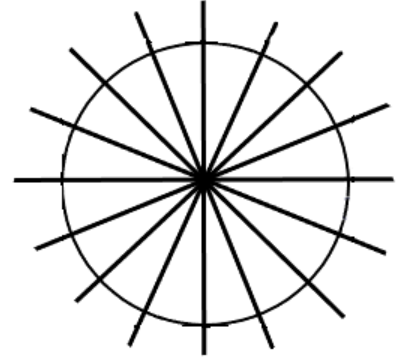There are infinite lines of symmetry. This line of symmetry is also known as the diameter of the circle.

## What will you learn in Class 7 Maths Chapter 14 Exercise 14.1 solutions of Vedantu?

Vedantu’s solutions are extremely easy to understand and creates an impact on the minds of the students. If you are a student of Class 7 CBSE board, then you must start studying from the Vedantu’s solutions.

Vedantu’s solutions contain all the topics of the Class 7 Maths Chapter 14.1 and provide you with a clear and concise idea of the different concepts that are taught in the chapter.

### Contents of the CBSE Class 7 Chapter 14 Maths Exercise 14.1

CBSE Class 7 Maths Exercise 14.1 consists of the following topics:

• Symmetry and its types

• Different shapes

• Centre of Rotation

• Order of Rotation

• Angle of Rotation

The questions that you will get answers to in the solution of Vedantu Class 7 Maths Chapter 14.1 are as follow:

1. State the numbers of the lines of symmetry in various figures

2. What letters of the English alphabet have reflectional symmetry?

3. What name can you give to the line of symmetry of different shapes ( isosceles triangle, circle, etc)?

4. Give examples of shapes with no lines of symmetry.

5. Other questions related to diagrams.

### Why Should You Refer To the Solutions of Vedantu to Study Maths Exercise 14.1 Class 7?

Vedantu’s solutions on Maths Exercise 14.1 Class 7 consists of all the information related to the concept of Symmetry. Once you start studying from the solutions of Vedantu, you will have a better understanding of the entire chapter. Now you will learn better thus scoring high marks in the examination. The solutions od Vedantu are properly provided to you with the help of diagrams and effective representations.

You will be able yo remember the solutions better, thus you will save time while writing the answers in your answer sheets during the examination. The solutions are highly effective for both your school examination and other competitive examination in the future. The solutions are written in a simple and easy manner for enhancing the process of revision.

The solutions are coupled with better tips and tricks, helping students score high marks. These tricks can help a student reach the top of the marks list in examination by simply helping them solve the maths problems in a much better and impressive manner.

The PDFs are provided to students for free. You can download them on your PCs or laptops by just visiting the website of Vedantu. Or you can also download NCERT Solutions Class 7 Maths Chapter 14 Exercise 14.1 PDF crafted by Vedantu on your mobile phones. All you need to do is download the Vedantu app.

### Why Vedantu?

Vedantu is the one-stop solution for all your exam preparation needs. It’s the best online tutor that you can ever come across. The solutions of Vedantu are prepared by highly qualified teachers and professors who have been teaching students of the CBSE board for many years.

They know what exactly the students lack. Everyone wants to get high scores, but either they don’t get time to revise all topics or they don’t get the time to revise all the topics before exams.

The NCERT Solutions for Class 7 Maths Chapter 14 Exercise 14.1 is prepared with proficiency to help students learn anything is the least time possible. The solutions are written in the most captivating manner to make sure that the interest of students grows on the subject effectively.

Once you start studying the NCERT Solutions for Class 7 Maths Chapter 14 ex 14.1 you will get to learn all the concepts of the chapter in a simple manner, without much hassle and effort. Vedantu makes the process of learning and revising easier for you. You will see the difference yourself once you start referring to the solutions of Vedantu. All you need to do is go through the solutions of Vedantu once or twice and then write them on your once. That’s it and you are absolutely prepared with the chapter for your upcoming examination. Download the PDF of Vedantu on Class 7 Maths Chapter 14 ex 14.1 and kickstart your preparation for the upcoming examination.SHARETWEETSHARESUBSCRIBE